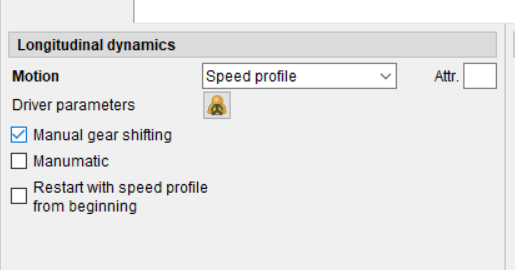# Longitudinal control of vehicle

Hello everyone,

I have two questions related to the longitudinal control of a vehicle using CarMaker.

1. Is there a way to deactivate the internal longitudinal controller within CarMaker? I aim to prevent CarMaker from changing the vehicle’s longitudinal acceleration.

2. If I only write the longitudinal acceleration value into Car.ax, will this suffice to ensure the vehicle attains the desired longitudinal acceleration? Essentially, to achieve a specific speed, I have designed a controller that only provides longitudinal acceleration (ax) values for the vehicle. However, I do not know how the gas and pedal should adjust to achieve this particular ax. How can I achieve this in CarMaker?

In short, my question is if I want the longitudinal value of the vehicle acceleration at time k to be ax=\alpha, just writing \alpha to Car.ax is going to be enough?

Kind regards,
Maryam

Hi MaryamN,

the longitudinal acceleration of a vehicle ax if the IPG driver is active can’t be changed since it is a calculation based on traction forces(vehicle powertrain power/torque) and resistive forces (rolling, aerodynamic, inertial forces). If you have the IPG Driver active as longitudinal manoeuvre you can limit the acceleration using the inputs below.

If you want to follow a speed profile (velocity that varies over time = acceleration) then I recommend using as longitudinal dynamics the speed profile as it is shown below.In this case the Driver will try to follow the speed profile (and therefore the acceleration profile), but, again, bear in mind that he will be able to follow if it is not limited by the traction forces(does the vehicle have sufficient power to accelerate to follow that speed?) or resistive forces.

I suggest you have a look at the speed profile section and follow some examples provided in the DrivingCycles example folder like the SC03 cycle below.